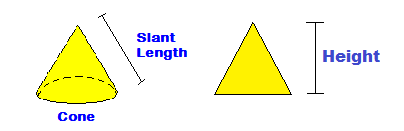CalcuNation

# Cone Volume Calculator

## Find the cone volume for math problems by using this Cone Volume Calculator.Cone Height:

Fill in the cone height and radius of the base to determine the volume.

### How do you find the volume of a cone?

The formula for the volume of a cone is: 1/3 x Pi x r2 x h

r = radius of the base

h = height of the cone

Pi = 3.1416

Example:

A cone with a height of 5 inches and a base radius of 3 inches has a
volume of:

1/3 x 3.1416 x 9 x 5 = 47.124 cubic inches

Other Calculators of Interest:
Circle Circumference Calculator, Sphere Area Calculator, Sphere Volume Calculator

### \$100 Promotion

Win \$100 towards teaching supplies! We want to see your websites and blogs.Enter Here

### Calculator Popups

Scientific Calculator
Simple Calculator

### Calculator Ideas

We use your calculator ideas to create new and useful online calculators.
Submit Calculator Idea